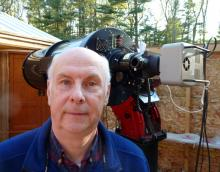# BSM_Hamren2 Transformation Coefficients

1 post / 0 new
MZKBSM_Hamren2 Transformation Coefficients

Attached are the transformation coefficients for Hamren2 which includes an E180 scope and an ASI183 CMOS camera. The std field images were collected on 200322.

SR is transformed to a Cousins R filter.

[Setup]
description= TG - Version TG_V7.4, Telescope= BSM_Hamren2, Time created (UT) = 2020_05_25_20:57:58
[Coefficients]
Tbv= 1.064
Tb_bv= 0.016
Tv_bv= -0.042
Tvr= 1.142
Tv_vr= -0.086
Tr_vr= -0.194
Tri= 0.860
Tr_ri= -0.218
Ti_ri= -0.041
Tvi= 0.986
Tv_vi= -0.044
Ti_vi= -0.018
Tr_vi= -0.106
[Error]
Tbv= 0.010
Tb_bv= 0.013
Tv_bv= 0.012
Tvr= 0.019
Tv_vr= 0.022
Tr_vr= 0.025
Tri= 0.017
Tr_ri= 0.029
Ti_ri= 0.038
Tvi= 0.014
Tv_vi= 0.012
Ti_vi= 0.018
Tr_vi= 0.013
[R Squared Values]
Tbv= 0.986
Tb_bv= 0.017
Tv_bv= 0.091
Tvr= 0.970
Tv_vr= 0.112
Tr_vr= 0.320
Tri= 0.965
Tr_ri= 0.314
Ti_ri= 0.012
Tvi= 0.979
Tv_vi= 0.099
Ti_vi= 0.010
Tr_vi= 0.323Hamren2 M67 200322 Transformation Coeff.txt